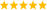# LabVIEW

cancel
Showing results for
Did you mean:

## Permute matrix columns to find the matrix with biggest trace

I am trying to make a Labview Vi that picks any 3x3 matrix and permutes the columns (not rows!) until it gets the ordenation that has a greatest trace (sum of diagonal elements). The program should also allow a sign change of the elements of one or more columns. If I am not wrong for a given 3x3 matrix and considering the column sign change there are 36 possible matrices, and the program should automatically show the one with greatest trace

For example:

1   6    3                                             6   -1   -3

-8  -2    4   should be converted to        -2    8   -4

3   2   -7                                             2   -3    7

I know it should be a quite simple program, but after spending several hours with L. V. 8.5  I end with a too complicated block diagram that does not work.

Ideally the program should also show of the permutation/sign change that has given the greatest trace but this part I think I am capable to do by myself.

Message Edited by obarriel on 12-28-2009 06:00 PM
Message 1 of 7
(2,819 Views)

## Re: Permute matrix columns to find the matrix with biggest trace

More brute force than anything else, but try this out as a starting point.

Message 2 of 7
(2,797 Views)

## Re: Permute matrix columns to find the matrix with biggest trace

Here's my version. It can easily be adapted to work with any size (within reason!) square matrix.

Since columns can be optionally negated, we can use the absolute value for the trace calculation so there are only 6 permutations to test in the case of a 3x3 array.

(I have a subVI that can generate a 2D array of all index permutations given N. We can replace the diagram constant with it and my VI will then work for random sized square input arrays.

See if this makes sense. 😉LabVIEW Champion. It all comes together inGCentralWhat does "Engineering Redefined" mean??
Message 3 of 7
(2,787 Views)

## Re: Permute matrix columns to find the matrix with biggest trace

Thank you very much to both of you!. I have tried both programs,

- Darin your vi does not output any matrix. At the moment I do not understand how it works, so I don't really see  where is the problem.

- C. altenbach I think there should be some issue with the program since it does not work properly for every matrix I input. In the attached program I put as default value one of my matrices that is not well-permuted.

Thank you very much again!

Oriol

Message 4 of 7
(2,776 Views)

## Re: Permute matrix columns to find the matrix with biggest trace

Funny.  It works for me (the version you reposted as well) and seems to give the answer you are looking for.  It may be a LV8.5 thing.  All I do is find different permutation matrices to shuffle and negate different columns, multiply and take the trace.  What part don't you understand?  I'll try to explain it (if you get it to work as is).Message 5 of 7
(2,764 Views)

## Re: Permute matrix columns to find the matrix with biggest trace

Sorry, I pasted the wrong permutation matrix diagram constant for some reason. As you can see, it has only two unique permutations instead of six. Silly! You can easily paste Darin's permutation matrix and it will work correctly. 😉

Here's a more general version that generates all permutations on the fly (LV 8.5). It does a 10x10 in under two seconds (3628800 permutations!).

See if this works better for you. 🙂

The permutation algorithm is from wikipedia:

For every number k, with 0 = k < n!, the following algorithm generates a unique permutation of the initial sequence sj, j = 1, ..., n:

function permutation(k, s) {
for j = 2 to length(s) {
swap s[(k mod j) + 1] with s[j]; // note that our array is indexed starting at 1
k := k / j;        // integer division cuts off the remainder
}
return s;
}
NOTE: Indexing in LabVIEW starts at 0, so the code is changed accordingly.

For larger matrices you'll run out of steam very quickly because the permutations increase with N factorial. I am sure there would be a much more

intelligent algorithm to solve all that in much less time. 🙂Message Edited by altenbach on 12-29-2009 09:31 AMLabVIEW Champion. It all comes together inGCentralWhat does "Engineering Redefined" mean??
Message 6 of 7
(2,758 Views)

## Re: Permute matrix columns to find the matrix with biggest trace

If moving beyond a fixed 3x3 matrix is the name of the game, hardwired constants and permutations are certainly not the way to go.  During some "private time" this afternoon I figured that I could tweak the classic assignment problem to solve this problem.  I had to do a quick conversion from my Scheme code (think Lisp) to LV, but it seems to work like a charm.  I use the well-known Munkres algorithm to find the solution for an arbitrary NxN matrix.  Since sign changes are allowed, I work with the absolute value of the matrix and at the end back out which columns are inverted based on their final position.  Since the Munkres algorithm is a cost minimazation technique, I negate the matrix so I get back the maximum.

Probably a few tweaks to improve memory management, etc., and maybe a bug or two but this is some quick and dirty code.  It will do a 200x200 matrix in about 2 seconds on my machine.  If I had a few trillion years on my hands I'd double check with the brute force method.

Message 7 of 7
(2,730 Views)